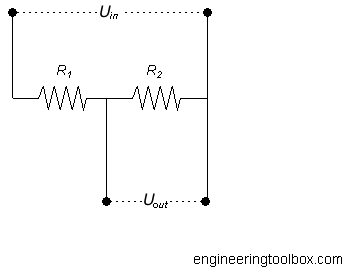Engineering ToolBox - Resources, Tools and Basic Information for Engineering and Design of Technical Applications!

# Potential Divider - Online Calculator

## Output voltage with a potential divider.

A potential divider is the simplest way of producing a source of lower e.m.f. from a source of higher e.m.f.The output voltage from a potential divider can be calculated as

Uout = Uin R2 / (R1 + R2)                 (1)

where

Uout = output voltage (V)

R = resistance (Ohms, Ω)

Uin = input voltage (V)

### Example - Potential Divider - High Power Consumption

The output voltage from a potential divider with two resistors R1 = 10 ohms and R2 = 20 ohms and input voltage 12 V can be calculated as

Uout = (12 V) (20 Ω) / ((10 Ω) + (20 Ω))

= 8 (V)

The current through the potential divider R1 and R2 (ex. output current) can be calculated by using Ohm's law

I = U / R

= (12 V) / ((10 Ω) + (20 Ω))

= 0.4 A

The power consumption of the divider can be calculated

P = U I

= (12 V) (0.4 A)

= 4.8 W

### Example - Potential Divider - Lower Power Consumption

The output voltage from a potential divider with two resistors R1 = 1000 ohms and R2 = 2000 ohms and input voltage 12 V can be calculated as

Uout = (12 V) (2000 Ω) / ((1000 Ω) + (2000 Ω))

= 8 (V)

The current through the potential divider R1 and R2 (ex. output current) can be calculated by using Ohm's law

I = U / R

= (12 V) / ((1000 Ω) + (2000 Ω))

= 0.004 A

The power consumption of the divider can be calculated

P = U I

= (12 V) (0.004 A)

= 0.048 W

The power consumption in a potential divider can be reduced by increased resistance.

### Potential Divider - Calculator

#### Potential Divider Nomogram

The nomogram below can be used to estimate a potential divider.

The default values in the nomogram abocve are for Uin = 12 VR2 = 47 ohm and Uout3.3 V. Since the sum of the resistances (R1 + R2) according the nomogram is approximately 170 ohm - the resistance R1 can be calculated as

R1 ≈ (170 ohm - 47 ohm)

≈ 123 ohm.

## Related Topics

• ### Electrical

Electrical units, amps and electrical wiring, wire gauge and AWG, electrical formulas and motors.

## Related Documents

• ### Current Divider - Online Calculator

An electric current divider outputs a current that is a fraction of the input current.
• ### Electrical Conductivity - Elements and other Materials

Electric conductance is the ability of a element to conduct an electric current.
• ### Electrical Parallel Circuits

Resistance, voltage and current in electrical parallel circuits.
• ### Electrical Resistance in Serial and Parallel Networks

Resistors in parallel and serial connections.
• ### Electrical Series Circuits

Voltage and current in electrical series circuits.
• ### Electromotive Force - e.m.f

Change in electrical potential between two points.
• ### Kirchhoff's Voltage and Current Laws

Kirchhoff's current and voltage laws.
• ### Resistance vs. Resistivity

Electrical resistance and resistivity.

## Engineering ToolBox - SketchUp Extension - Online 3D modeling!

Add standard and customized parametric components - like flange beams, lumbers, piping, stairs and more - to your Sketchup model with the Engineering ToolBox - SketchUp Extension - enabled for use with older versions of the amazing SketchUp Make and the newer "up to date" SketchUp Pro . Add the Engineering ToolBox extension to your SketchUp Make/Pro from the Extension Warehouse !

We don't collect information from our users. More about

## Citation

• The Engineering ToolBox (2008). Potential Divider - Online Calculator. [online] Available at: https://www.engineeringtoolbox.com/potential-divider-d_1384.html [Accessed Day Month Year].

Modify the access date according your visit.

10.2.10